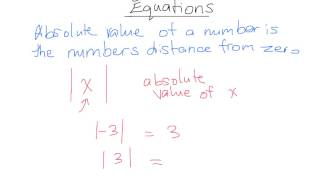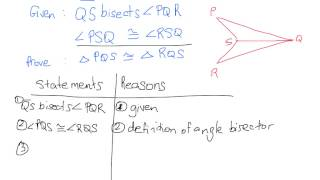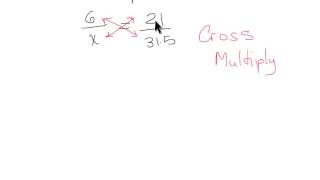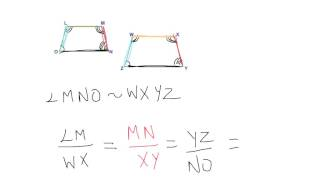## Nicole HamiltonNicole Hamilton

## User videosSolving Absolute Value Equations
This video describes what an absolute value is then goes over how to solve an absolute value equation.Proving Triangle Congruence (Part 4)
This lesson will look at an example proving triangle congruence using the angle side angle postulateRatios and Proportions in Geometry
This lesson will look at an example on how to work with ratios and proportions in geometry.Similar Polygons
This lesson will look at what is a similar polygon? How can they be identified? How do we find an unknown sides in similar polygons?